# Past paper Questions i stated to do but cant complete xD

Status
Not open for further replies.

#### xSimon_B

##### Newbie level 1Past paper Questions i started to do but cant complete xD

Please note!
• I am not looking for answers
• I am looking for help/tips on what to do
• I do not know if this is the right forum for my post (the mathematics + physics) bit, and if it is not the right place for my place i would be happy if the thread was moved to the right place. ThanksAlright a little about myself? ;D

I go to college studying a HND in electronic engineering but it seems my ability to learn new things isnt as great as i once thought... either that or the notes i have are rubbish... although they are no help so i shall blame the notes ^^

Alright so my problems atm are probably simple to you guys lol!

Anyways im here for, not realy help, but to see if my answeers are correct since this past paper doesnt have an answer sheet which is really unfair Dx

anyways Q1 was to do with sequential logic and i have got that right because sequential log is my favourite lol!

Q2: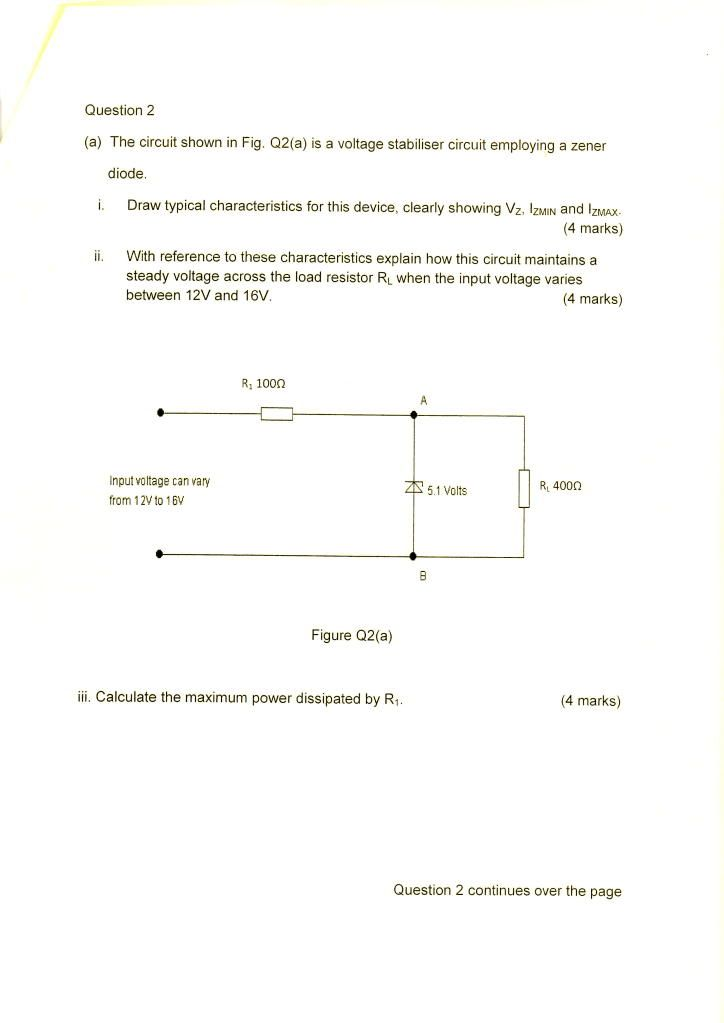So my answers here is a + shaped graph and the line is the shape of ,-' (hard to show lol)
part II) The Zener is reverse baised so it will limit the voltage to 5.1v flowing through it and allow current to flow through

iii. didn't know left blank 0 marks lol

Question 2b is calculating a J-fet transistor values and i havn't covered that yet so i left that blank.

Question 3: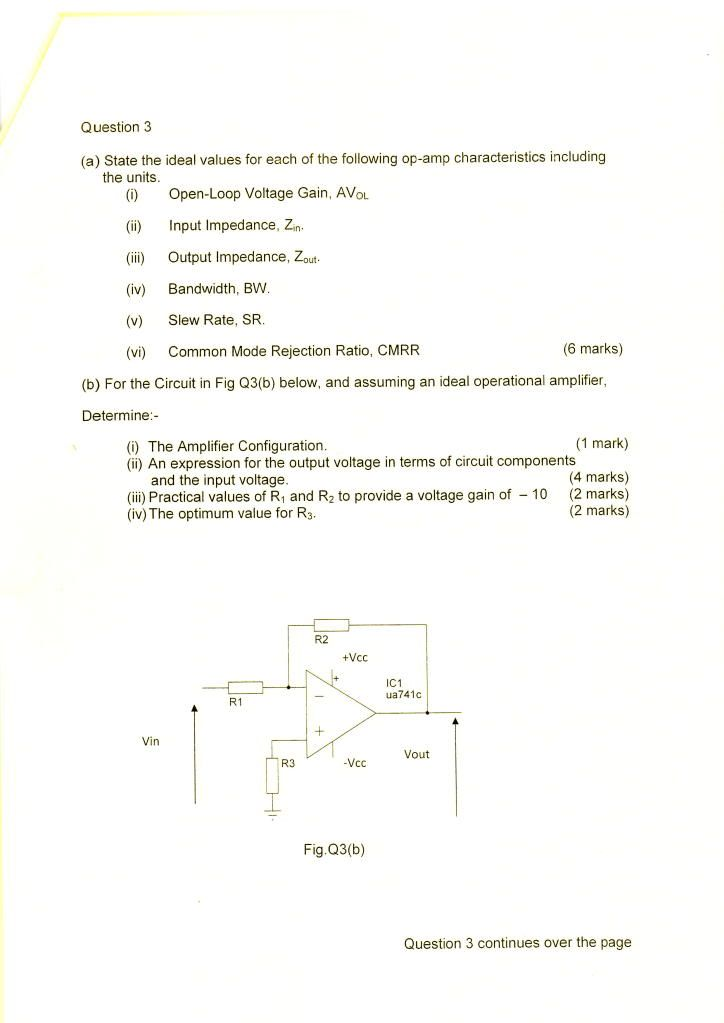a i) Infinite
ii) Infinite
iii) Zero
iv) infinite
v) Zero
vi) Zero

3b) Non inverting op-amp
ii) Vo = Vin + I2 x R2
iii) Don't know
iv) Don't know

Question 3c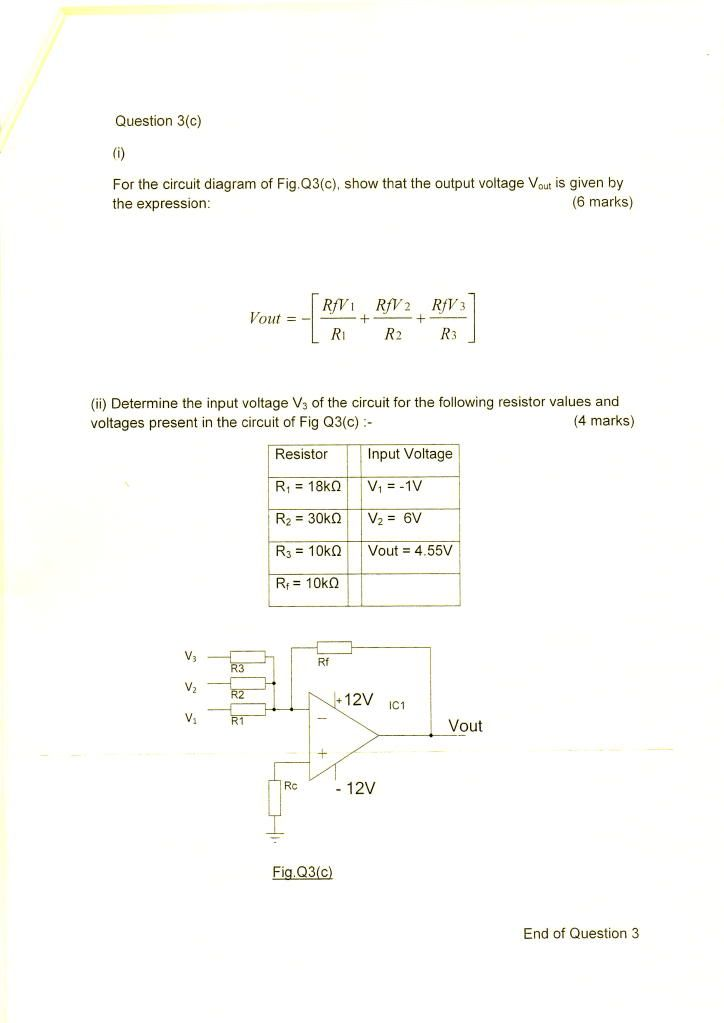I was able to show the the expression as

If = I1+I2+I3 = -[v1/r1 + v2/r2 + v3/r3]

Vout = -(rf/r123) x Vin

- Vout = [ Rfv1/r1 + Rfv2/r2 Rfv3/r3]

ii) Dont know how to do this yet we will be taught this i have been told xD

Question 4 and 5 and 5b are the ones i was looking for most help on.

Question 5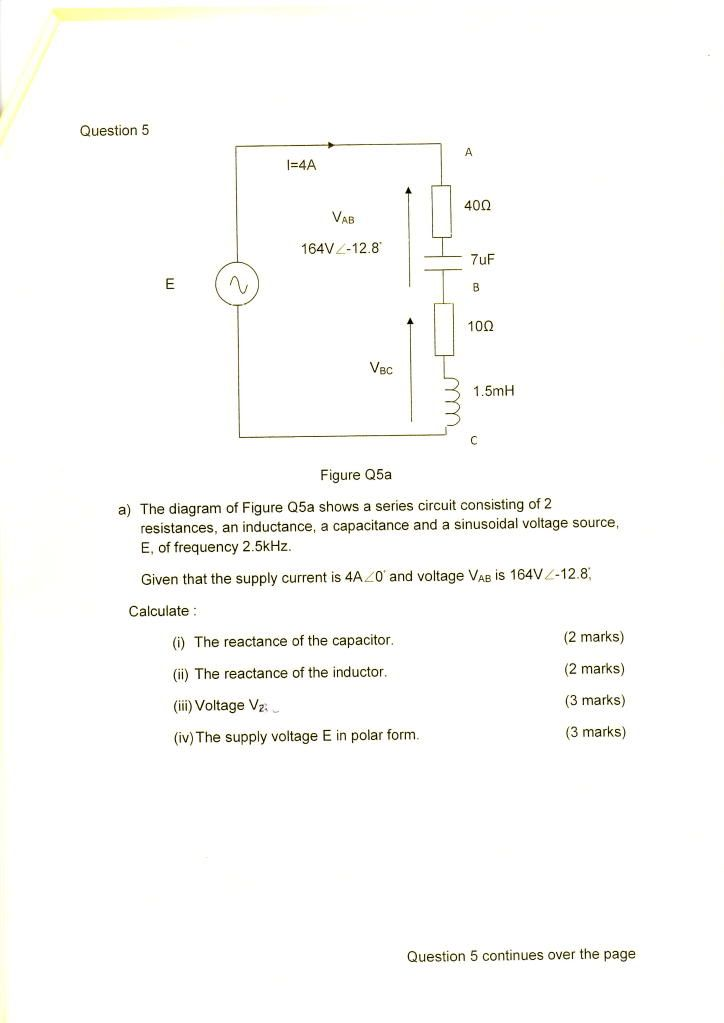i) So i calculated xc by using 1/2 * pi * f * c
= 1/2 * pi * 2.5k * 7uF
Xc = 27.49 Ω

ii) and XL was 2 * pi * F * L
= 2 * pi * 2.5k * 1.5mH
XL = 23.56

iii) Vbc -> V = I * R
= 4A * (10 + J23.56)
= 4 * 25 <67°

Vbc = 100 <67 (Now conv to polar)
= 39+J92.1

iv) To get E i should add Vab + Vbc therefore

Vab = 164 <-12.8 (now convert to rec) = 159-J36.33
Vbc = 39 + J92.1

Add ab + bc = 39+j92.1 + 159-j36.33 = 198+j57.77

To pol = 205 <15.7°

Which i think is right xD

5b i have no idea how to work out part IV and V so if you could give me hand there that would be awesome ^^

Alright so this is my last question

question 5b: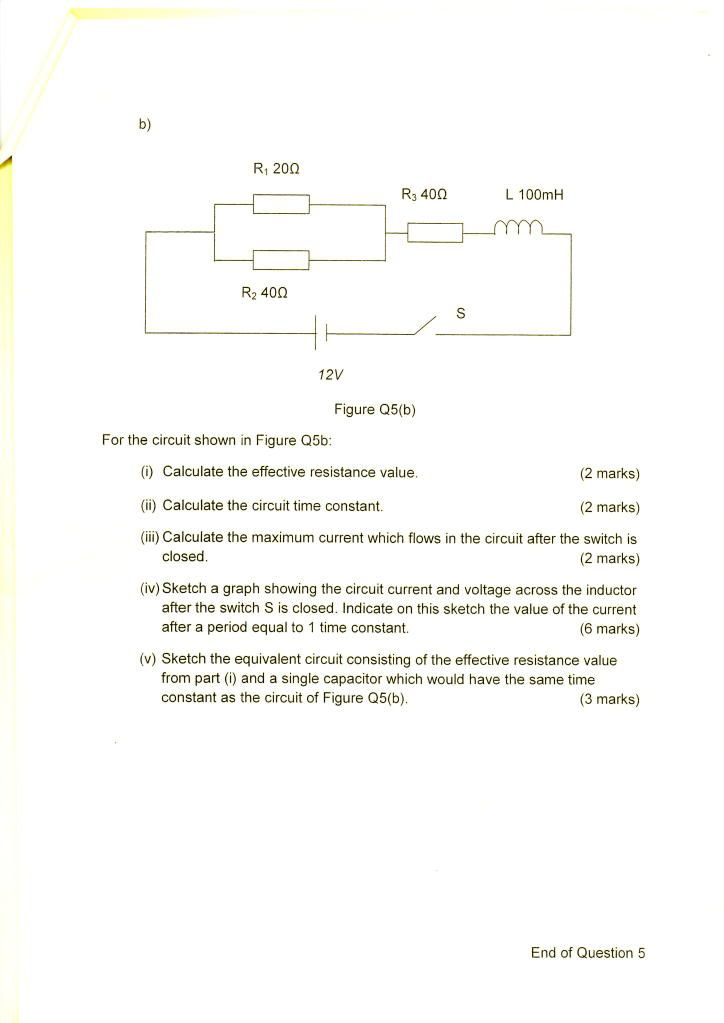I worked out (i) with r1xr2 / r1 + r2 = 13.33 Ω + 40Ω = Rt 53.33Ω

I worked out (ii) by doing T = L/R = 100x10-3 / 53.333 = 1.88ms
^ not 100% sure this is right?

(iii) I worked out the maximum current flowing as
I = V/R = 12 / 53.33 = 0.23A

part (iv) I do not know how to do... The graph must be |_ and it rises and curves until the max current is reached? I on the y axis, T on the x axis?

v i have no clue how to do so no need to explain that one to me ill ask my tutor lol.

I know its alot to ask but im not asking that you answer every question for me all i ask is that, if possible, could you read over my answers and tell me if im right or wrong. If i am wrong you don't need to give me the answer but a small bit of help would be very much appreciated.

Thanks to all who help
Simon.Forgot this question

Question 4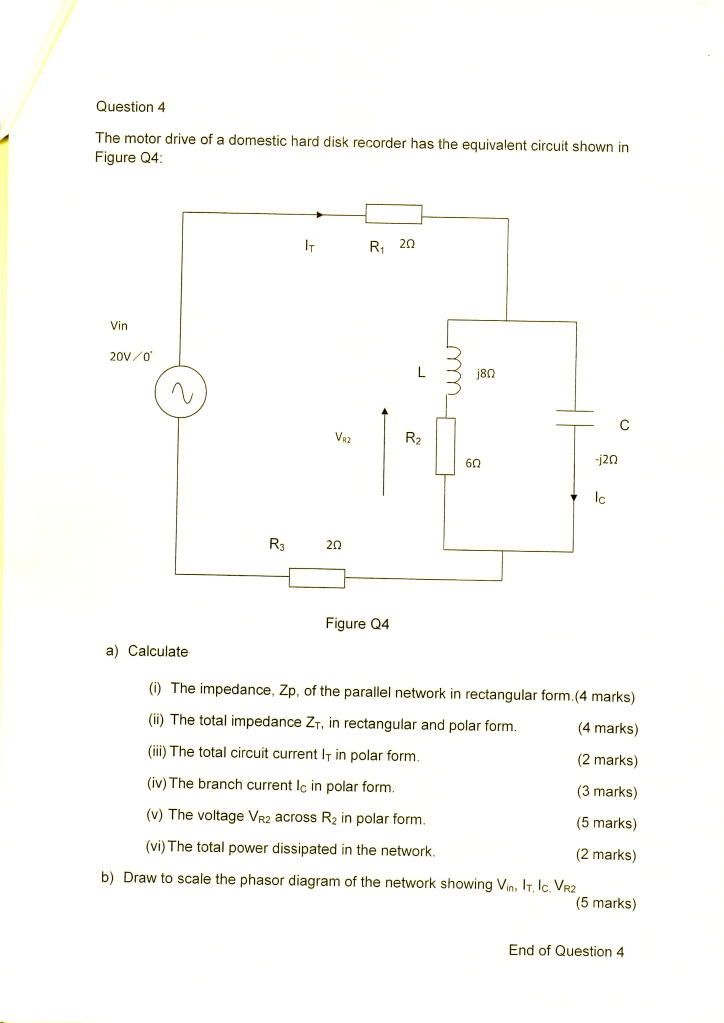I have no idea how to do this question so any help is appreciated xD

do i use L and R2 as 6 + J8 then use C (-J2) and turn it into R1 = 2 (parrallel bit = 6+J6) R3 = 2 and use that for the rest of the question? :S

Last edited:

Status
Not open for further replies.